# 10.3 Discussion and Exercises

The implicit representation of a complete binary tree as an array, or list, seems to have been first proposed by Eytzinger . He used this representation in books containing pedigree family trees of noble families. The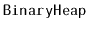data structure described here was first introduced by Williams .

The randomized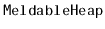data structure described here appears to have first been proposed by Gambin and Malinowski . Other meldable heap implementations exist, including leftist heaps [16,48, Section 5.3.2], binomial heaps , Fibonacci heaps , pairing heaps , and skew heaps , although none of these are as simple as thestructure.

Some of the above structures also support aoperation in which the value stored at nodeis decreased to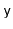. (It is a pre-condition that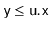.) In most of the preceding structures, this operation can be supported in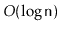time by removing node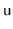and adding. However, some of these structures can implement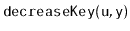more efficiently. In particular,takes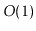amortized time in Fibonacci heaps and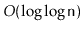amortized time in a special version of pairing heaps . This more efficientoperation has applications in speeding up several graph algorithms, including Dijkstra's shortest path algorithm .

Exercise 10..1   Illustrate the addition of the values 7 and then 3 to theshown at the end of Figure 10.2.

Exercise 10..2   Illustrate the removal of the next two values (6 and 8) on theshown at the end of Figure 10.3.

Exercise 10..3   Implement the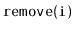method, that removes the value stored in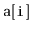in a. This method should run intime. Next, explain why this method is not likely to be useful.

Exercise 10..4   A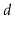-ary tree is a generalization of a binary tree in which each internal node haschildren. Using Eytzinger's method it is also possible to represent complete-ary trees using arrays. Work out the equations that, given an index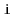, determine the index of's parent and each of'schildren in this representation.

Exercise 10..5   Using what you learned in Exercise 10.4, design and implement a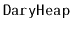, the-ary generalization of a. Analyze the running times of operations on aand test the performance of yourimplementation against that of theimplementation given here.

Exercise 10..6   Illustrate the addition of the values 17 and then 82 in the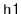shown in Figure 10.4. Use a coin to simulate a random bit when needed.

Exercise 10..7   Illustrate the removal of the next two values (4 and 8) in theshown in Figure 10.4. Use a coin to simulate a random bit when needed.

Exercise 10..8   Implement themethod, that removes the nodefrom a. This method should run inexpected time.

Exercise 10..9   Show how to find the second smallest value in aorin constant time.

Exercise 10..10   Show how to find theth smallest value in aorin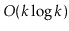time. (Hint: Using another heap might help.)

Exercise 10..11   Suppose you are given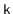sorted lists, of total length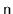. Using a heap, show how to merge these into a single sorted list in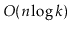time. (Hint: Starting with the case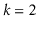can be instructive.)

opendatastructures.org